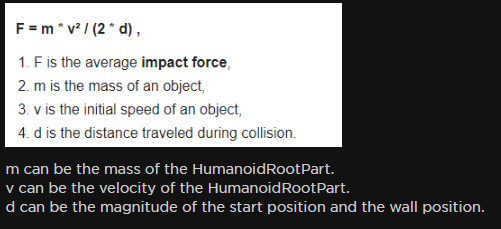# How would i get the "Starting Position"

Looking through posts for a answer to get impact force realistcly (somewhat)
and found this(Detecting when a flung character has hit a wall - #4 by Dav_itt)
i already got m and v down, just trying to get d (the magnitude between the start position and the hit position)

While i can get the end / hit position, im not too sure how i would get the starting position

Heres what i currently got down

``````local ExplosivePart = script.Parent
local Nm = 10

local Vx = 0
local Vy = 0
local Vz = 0

local Distance

local Gst = false
local Get = false

local StartTime = tick()
local EndTime = tick()

local HitVelocity

local LastHit
local LastVelocity

local function A_1()
local V = ExplosivePart.AssemblyLinearVelocity
Vx = if V.X > 0 then V.X else 0
Vy = if V.Y > 0 then V.Y else 0
Vz = if V.Z > 0 then V.Z else 0

end

local function A1()
local V = ExplosivePart.AssemblyLinearVelocity

if Vy > 10 or Vx > 10 or Vz > 10 then
if not Gst then StartTime = tick(); Gst = true end
end

if Get and Gst then
local Vctr = (Vx+Vy+Vz)
end
end
end

A1()

ExplosivePart:GetPropertyChangedSignal("AssemblyLinearVelocity"):Connect(A_1)
ExplosivePart.Touched:Connect(function(Part)

end)
``````

(edit)
Updated code

I’m not great at math, but couldn’t you just store the current speed of the object upon hit?. Since its like `Studs Per Second` then you can use that as the distance traveled during collision, not too sure thought since I feel like if you have 9999999 acceleration and you went 0-100 without really moving much and you hit someone that could lead into a insta kill, assuming this is for like ramming damageCouldn’t you store the start position at the start of A1?

`local VStart = ExplosivePart.CFrame`

Then just refer to VStart once you hit your target, and calculate magnitude from there.

.touched doesnt work in my favor most of the time, tried using it once on a conveyor and it just kept spamming the .touched event

No, kinda like gmod explosive barrels if they hit something hard enough they explode

yeah but what if it is moved around before it gets launched or thrown off a cliff?
the starting position would be way farther than its real position from where it got thrown

A1 gets called when it is thrown, correct?

not really, A1 is just called as of right now
tried another method of getting it

``````local ExplosivePart = script.Parent
local Nm = 10

local Vx = 0
local Vy = 0
local Vz = 0

local Distance

local Gst = false
local Get = false

local StartTime = tick()
local EndTime = tick()

local HitVelocity

local LastHit
local LastVelocity

local function A_1()
local V = ExplosivePart.AssemblyLinearVelocity
Vx = if V.X > 0 then V.X else 0
Vy = if V.Y > 0 then V.Y else 0
Vz = if V.Z > 0 then V.Z else 0

end

local function A1()
local V = ExplosivePart.AssemblyLinearVelocity

if Vy > 10 or Vx > 10 or Vz > 10 then
if not Gst then StartTime = tick(); Gst = true end
end

if Get and Gst then
local Vctr = (Vx+Vy+Vz)
end
end
end

A1()

ExplosivePart:GetPropertyChangedSignal("AssemblyLinearVelocity"):Connect(A_1)
ExplosivePart.Touched:Connect(function(Part)

end)
``````

thought i could get the “time it got thrown or pushed off a edge” by just checking if its velocity was high enough.

while this (kinda, but not really) works not sure how to get end time accurately now using this method

had another idea to this type of method, if the part gets hit / on hit save the speed, and then check like .1 or a tick() later and check if the velocity dropped down or something.

then realized you can have negative velocity and this probably wouldn’t work

You’re trying to find `d` right?
solving for `d` it would be: `d = (m * v ^2) / (2 * f)`, where f /= 0

So basically `Distance = (Mass * Velocity^2) / (2 * ?)` not sure where i get f

Got it figured out

``````local ExplosivePart = script.Parent
local ToCancelFall = ExplosivePart.CancelFall.Value

local Touched = false
local Debug = false
local Vx = 0
local Vy = 0
local Vz = 0

local StartedFall = false
local EndedFall = false

local StartTime = tick()
local EndTime = tick()

local StartPos = Vector3.zero
local TouchedPos = Vector3.zero

local Force

ExplosivePart.Touched:Connect(function(Part)
Touched = true
TouchedPos = ExplosivePart.Position
end)

local V = ExplosivePart.AssemblyLinearVelocity

local X1 = if math.abs(V.X) > 0 then math.abs(V.X) else 0
local Y1 = if math.abs(V.Y) > 0 then math.abs(V.Y) else 0
local Z1 = if math.abs(V.Z) > 0 then math.abs(V.Z) else 0

if X1 > Vx then Vx = X1 end
if Y1 > Vy then Vy = Y1 end
if Z1 > Vz then Vz = Z1 end

if Vy > 10 or Vx > 10 or Vz > 10 then
if not StartedFall then StartTime = tick(); StartPos = ExplosivePart.Position; StartedFall = true end
end

if StartedFall then
if Touched then
local hit = false
local V2 = ExplosivePart.Velocity

if Vx - V2.X > Vx * .20 then hit = true end
if Vy - V2.Y > Vy * .20 then hit = true end
if Vz - V2.Z > Vz * .20 then hit = true end

if hit == true then
EndTime = tick()

local Vctr = (Vx+Vy+Vz)^2
local Mass = ExplosivePart.Mass
local Distance = (TouchedPos - StartPos).Magnitude

if Distance < 10*(Mass/3) then Distance = 10*(Mass/3) end
Force = (Mass * Vctr / (2 * Distance))/100

print(Force)
if Force >= 5 then
ExplosivePart:FindFirstChildWhichIsA("Humanoid"):TakeDamage(Force)
end

Vx = 0
Vy = 0
Vz = 0

Distance = nil
StartedFall = false
Touched = false
hit = false

if Debug then
print("Part fell for " .. EndTime - StartTime .. " Seconds")
end
else
Touched = false
print("false Signal")
end
end
end
end

``````